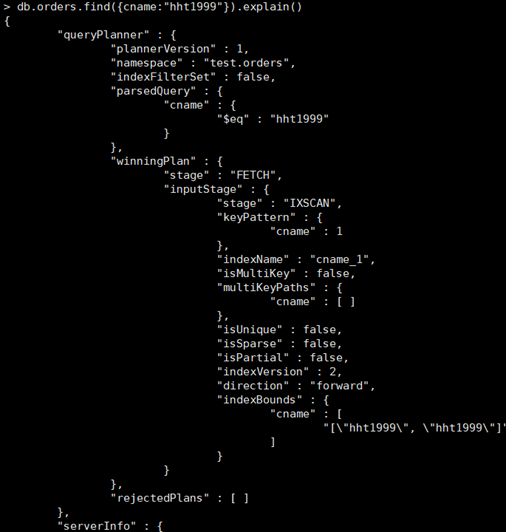## 在MongoDB中执行查询与创建索引

（1）掌握MongoDB中数据查询的方法；
（2）掌握MongoDB中索引及其创建；

（1）find函数的使用；
（2）条件操作符： $all匹配所有、$exists判断字段是否存在、null值处理、$mod取模运算、不等于、包含、不包含、数组元素个数、限制返回、排序、分页、随机显示。 （3）distinct找出给定键所有不同的值； （4）group分组； （5）游标； （6）存储过程。 二、 MongoDB中索引及其创建； （1）基础索引； （2）文档索引； （3）组合索引； （4）唯一索引； （5）强制使用索引； （6）扩展索引； （7）删除索引 （8）explain执行计划 ### 一、MongoDB中数据查询的方法 #### (1). find函数的使用 db.student.find() 查询所有数据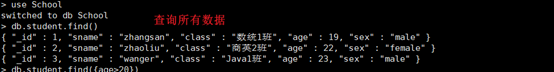#### （2）. 条件操作符$all匹配所有、
db.student.find({sex:{$all:['male']}}) 查询男生的学生数据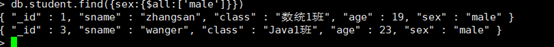$exists判断字段是否存在
> db.student.find({class: {$exists:true}}) 查询所有拥有class字段的学生信息；如果exists:false，表示不存在这个字段的信息。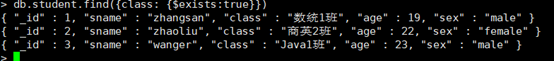null值处理 teacher集合中，_id=1的数据没有字段age,或者说age字段对应的值是null，所以可以进行null值处理，将他查出来。 db.teacher.find({age:null})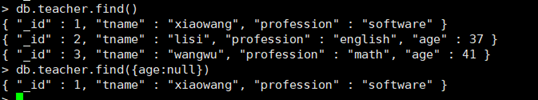$mod取模运算

db.teacher.find({age:{$mod:[10,1]}})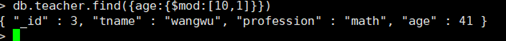运算符： -$gt 大于
- $gte 大于等于 -$lt 小于
- $lte 小于等于 -$ne 不等于
- $in 包含 -$nin 不包含
- $eq 等于 查询学生年龄大于22的数据 db.student.find({age:{$gt:22}})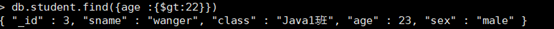db.student.find({age:{$lt:22}})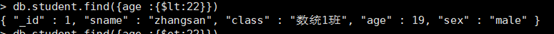查询学生年龄等于22的数据 db.student.find({age:{$eq:22}})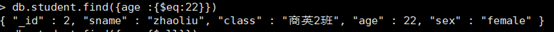db.student.count()#查出student集合中的记录数
db.student.find({sex:'male'}).count()#查出student集合中sex=male的人数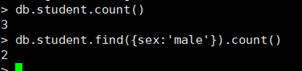db.student.find({},{sname:1}) # 返回sname字段其余不返回（id也返回）
db.student.find({},{sname:0}) # 除sname字段不返回外，其余都返回。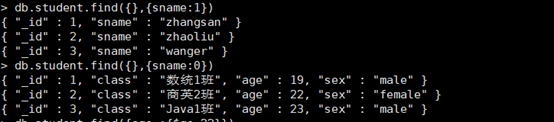db.student.find().sort({age:-1})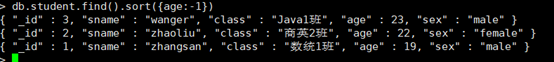db.student.find().sort({age:1})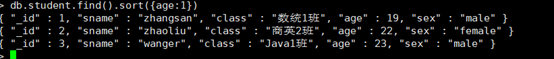db.student.find().limit(2)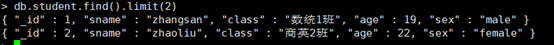db.student.aggregate([ { $sample: { size: N } } ] ) 从学生表中随机取出N条数据。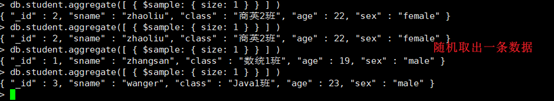### （3）distinct找出给定键所有不同的值； db.student.distinct(‘sname’) #找出不同的名字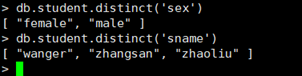### （4）group分组 求出年龄总和 db.student.aggregate( [ {$group: {
_id: null,
total: { $sum: "$age" }
}
}
] )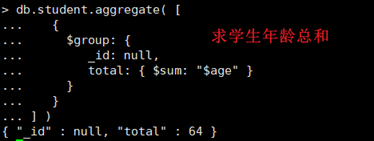db.student.aggregate([
{
$group: { _id: null, total: {$avg: "$age" } } } ])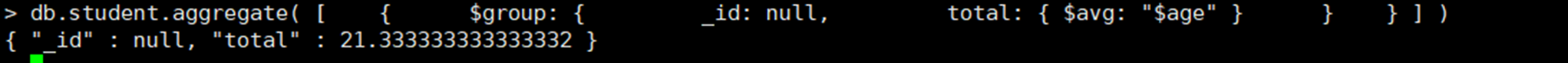### （5）游标； 定义游标 var mycursor = db.student.find()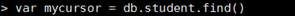打印结果 while(mycursor.hasNext()){ printjson(mycursor.next()); }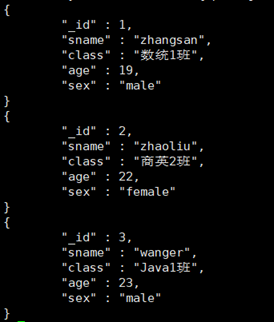也可以使用游标的forEach(printjson); 迭代访问文档.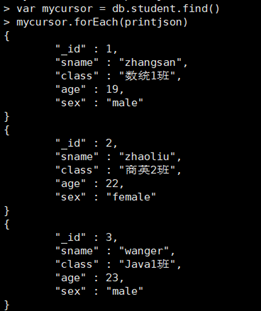显示游标状态信息 > db.serverStatus().metrics.cursor { "timedOut" : NumberLong(0), "open" : { "noTimeout" : NumberLong(0), "pinned" : NumberLong(0), "total" : NumberLong(0) } }  • timedOut：自服务器器启动以来超时游标数; • noTimeout：使用DBQuery.Option.noTimeout 选项防止超时打开的游标数; • pinned ：打开的游标数量; • total：打开游标的总数量; 关闭不活跃的游标 如果一个游标还没有遍历完，但是不使用了，需要将该游标关闭。默认情况下，服务器会自动关闭超过10分钟活跃的游标和还未耗尽的游标。你也可以自己手动关闭。 手动关闭前：var mycursor = db.student.find().noCursorTimeout(); 然后在 cursor.close()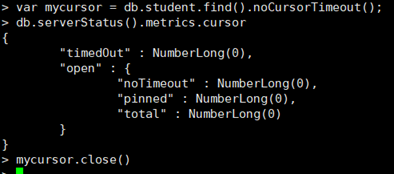### （6）存储过程。 MongoDB 存储过程是存储在 db.system.js 表中的. 1、 添加存储过程 db.system.js.save ( { _id:"getStuCount", value:function(){ return db.student.find().count(); }, description:"获取总数" } )  执行存储过程：var obj = db.eval(“getStuCount()”);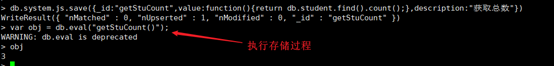2、 查询存储过程 db.system.js.find();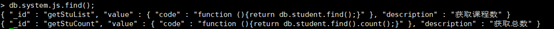3、 修改存储过程 先添加一个add的存储过程 db.system.js.save({_id:“add”,value:function(x,y){return x+y;}})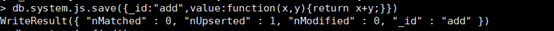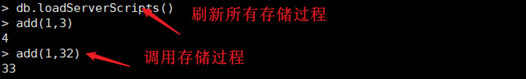修改add存储过程： db.system.js.update( {_id:"add"}, {$set:{value:function(x,y,z){return x+y+z;}}}
);


db.loadServerScripts()：重新加载存储过程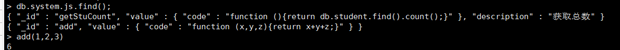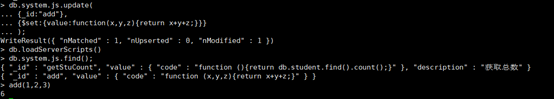4、 删除存储过程

db.system.js.remove({_id:‘getStuList’});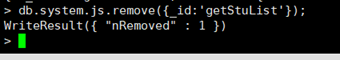### MongoDB中索引及其创建

db. orders.insert({
"onumber" : i,
"date" : "2020-06-09",
"cname" : "hht"+i,
"items" :[ {
"ino" : i,
"quantity" : i,
"price" : 4.0
},{
"ino" : i+1,
"quantity" : i+1,
"price" : 6.0
}
]
})


（1）基础索引；

db.orders.getIndexes()查看orders文档的索引信息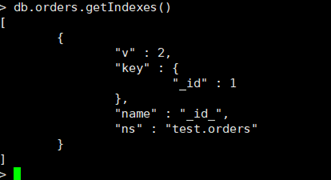db.orders.createIndex({cname:1})
{
“createdCollectionAutomatically” : false,
“numIndexesBefore” : 1,
“numIndexesAfter” : 2,
“ok” : 1
}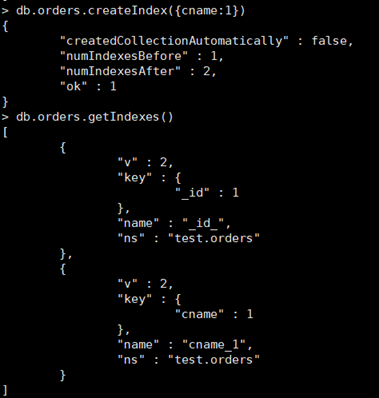（2）文档索引；

db.orders.createIndex({“item.info”:1})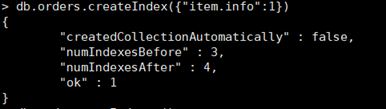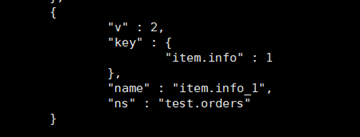（3）组合索引；

db.orders.createIndex({cname:1,onumber:-1})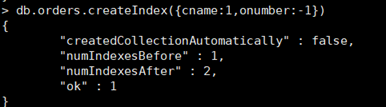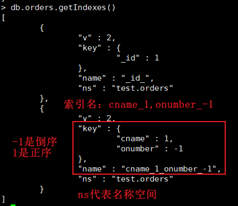（4）唯一索引；

db.orders.createIndex({onumber:1},{unique:true})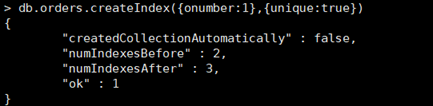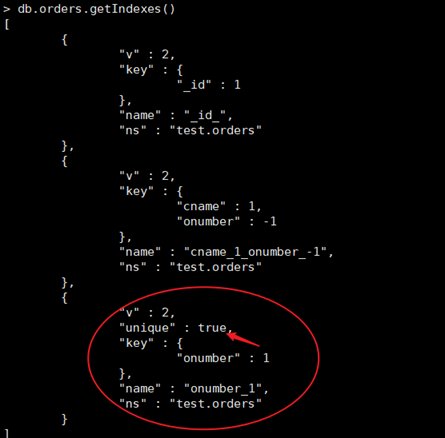（5）强制使用索引；

db.orders.find({“cname”:{$gt:“hht1000”},“onumber”:2000}).hint({onumber:1}) 这里是强制使用 onumber字段上的索引。 对这个查询执行explain() db.orders.find({"cname":{$gt:"hht1000"},"onumber":2000}).hint({onumber:1}).explain()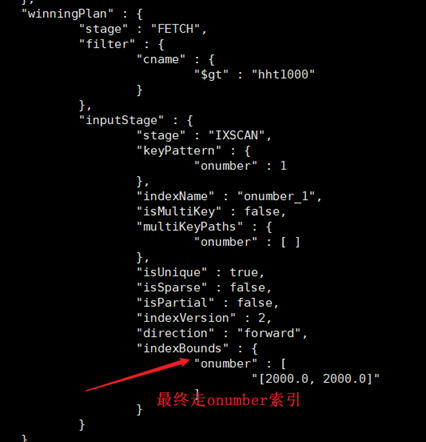（6）扩展索引；

（7）删除索引

> db.orders.dropIndex("cname_1")
{ "nIndexesWas" : 2, "ok" : 1 }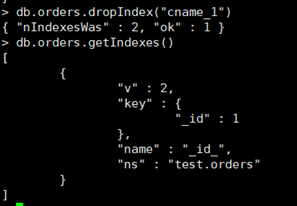db.orders.dropIndexes()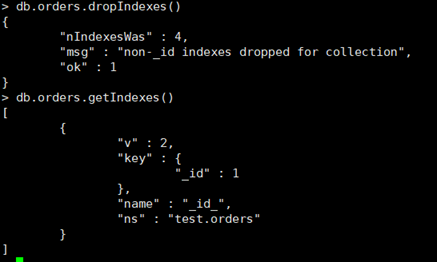（8）explain执行计划